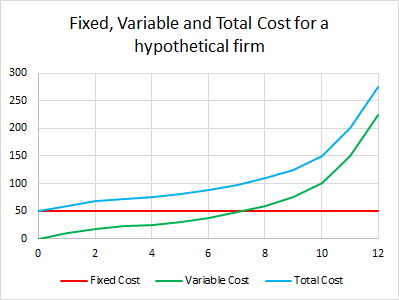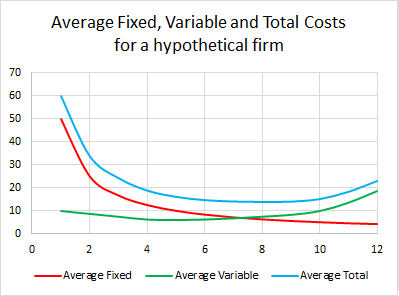# Costs for a firmFigure 1. A graph showing how the fixed costs stay constant, the variable costs change and the total cost is the sum of the two.Figure 2. A graph showing how the average fixed cost, the average variable cost change with units produced. Note how the variable cost decreases up to a point, but with more units produced, the average variable cost starts to increase again.

Firms have a number of different types of costs including fixed cost, variable cost, total cost and marginal cost. All of these different costs need to be considered when looking to figure out the costs to a firm. Figure 1 shows how the individual costs change with the level of output of a firm, check a cost column from the table (at the bottom of the page) and compare with the corresponding line on the graph to see what each cost does with increased output. Of course, the more units produced, the more units can be sold. This means that the average costs should be considered. As seen in figure 2, the fixed costs for production drop with more units produced, while the variable and total costs have a slightly more complicated relationship. Both figure 1 and figure 2 come from the data in the table below.

• Fixed Cost: The cost that is incurred regardless of the number of units produced. Note that the fixed costs have to be paid, even with no production.
• Variable Cost: The cost that accounts for the cost of production, excluding what is covered in the fixed costs.
• Total Cost: The fixed plus variable costs.

Within these costs are other types of cost as well:

• Accounting Cost: This is the operation cost of running a business combined with the cost of inputs such as raw materials, labour and rent.
• Opportunity Cost: This is the cost of using an input to do one thing instead of another of thing of value. For example a petrochemical company might have a reserve of crude oil that they keep to cope with spikes in demand or short falls in supply. The opportunity cost of holding the crude oil out of the market is the value that the oil could be sold to another firm for.
• Economic Cost: This cost is the accounting costs of a firm combined with its opportunity cost.

This table shows how the various costs interact for a hypothetical firm.

Output
Quantity
Fixed Cost
FC
Variable Cost
VC
Total Cost
TC
Marginal Cost
MC
Average Fixed
Cost
AFC
Average Variable
Cost
AVC
Average Total
Cost
ATC
0 50 0 50 - - - -
1 50 10 60 10 50 10 60
2 50 17.5 67.5 7.5 25 8.75 33.75
3 50 22.5 72.5 5 16.67 7.5 24.17
4 50 25 75 2.5 12.5 6.25 18.75
5 50 30 80 5 10 6 16
6 50 37.5 87.5 7.5 8.33 6.25 14.58
7 50 47.5 97.5 10 7.14 6.79 13.93
8 50 60 110 12.5 6.25 7.5 13.75
9 50 75 125 15 5.56 8.33 13.89
10 50 100 150 25 5 10 15
11 50 150 200 50 4.55 13.64 18.18
12 50 225 275 75 4.17 18.75 22.92